Definition: Odds Ratio from Dictionary of Nursing Theory and Research

An odds ratio is a summary index usually associated with the results of a case-control study, and is calculated by dividing the odds of exposure for the cases who contract the disease by the odds of exposure for the controls who do not contract the disease (or occasionally the other way around, i.e., the odds for the controls divided by the odds for the cases). It is a good approximation to the relative risk for rare diseases.

Summary Article: Odds Ratio
From Encyclopedia of Research Design

The odds ratio (OR) is a measure of association that is used to describe the relationship between two or more categorical (usually dichotomous) variables (e.g., in a contingency table) or between continuous variables and a categorical outcome variable (e.g., in logistic regression). The OR describes how much more likely an outcome is to occur in one group as compared to another group. ORs are particularly important in research settings that have dichotomous outcome variables (e.g., in medical research).

As the name implies, the OR is the ratio of two “odds,” which are, in turn, ratios of the chance or probability of two (or more) possible outcomes. Suppose one were throwing a single die and wanted to calculate the odds of getting a 1, 2, 3, or 4. Because there is a 4 in 6 chance of throwing a 1 through 4 on a single die, the odds are 4/6 (the probability of getting a 1 through 4) divided by 2/6 (2/6 is the probability of not getting a 1 through 4, but rather getting a 5 or 6) or 4/2 = “2 to 1” = 2.

An Example: Odds Ratios for Two Dichotomous Outcomes

David P. Strachan, Barbara K. Butland, and H. Ross Anderson report on the occurrence of hay fever for 11-year-old children with and without eczema and present the results in a contingency table (Table 1).

First, the probability of hay fever for those with eczema (the top row) is calculated. This probability is 141/561 = .251. Thus, the odds of hay fever for those with eczema are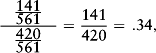about 1 to 3. Analogously, the probability of hay fever for those without eczema is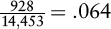, and the odds are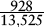. The OR in this example is defined as the odds of hay fever for eczema patients divided by the odds of hay fever for non-eczema patients. The OR, therefore, is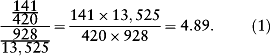From the example, one can infer that the odds of hay fever for eczema sufferers are 4.89 times the odds for noneczema patients. Thus, having eczema almost quintuples the odds of getting hay fever.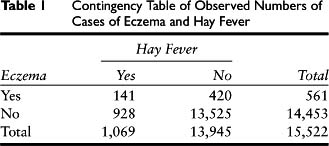The ratio of the two odds can, as shown in Equation (1), also be computed as a ratio of the products of the diagonally opposite cells and is also referred to as the cross-product ratio.

The OR is bounded by zero and positive infinity. Values above and below 1 indicate that the occurrence of an event is more likely for one or the other group, respectively. An OR of exactly 1 means that the two odds are exactly equal, implying complete independence between the variables.

Does the Odds Ratio Imply a Significant Relationship?

To determine whether or not this OR is significantly different from 1.0 (implying the observed relationship or effect is most likely not due to chance), one can perform a null hypothesis significance test. Usually, the OR is first transformed into the log odds ratio [log(OR)] by taking its natural logarithm. Then, this value is divided by its standard error and the result compared to a test value. In this example, the OR of 4.89 is transformed to the log(OR) of 1.59. A log(OR) of 0 implies independence, whereas values further away from 0 signify relationships in which the probability or odds are different for the two groups. Note that log(OR) is symmetrical and is bounded by negative and positive infinity.

The standard error (SE) of the log(OR) is defined as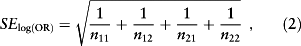where n11 to n22 are sample sizes of the four cells in the contingency table. Using this formula, the SElog(OR) in the example is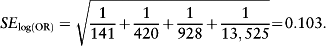Thus,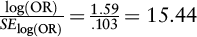.

In large samples, the sampling distribution of log(OR) can be assumed to be normal and thus can be compared to the critical values of the normal distribution (that is, with α = .05, the values +/− 1.96). Because the obtained value far exceeds this critical value, the OR can be said to be significantly different from 1 at the .05 level.

Alternatively, one can use the confidence interval approach. The 95% confidence interval for this example's log(OR) is 1.59 +/- (0.103)(1.96) and thus ranges from 1.386 to 1.790. The result of the previous significance test is reflected here by the fact that the interval does not contain 0. Alternatively, the 95% confidence interval around the OR [rather than the log(OR)] can be found. To do so, one takes the antilog of the confidence limits of the log(OR) interval, yielding a confidence interval around the OR estimate of 4.89 that ranges from 4.00 to 5.99. Because the OR was shown earlier to be statistically significant, the interval will not contain 1.0. Note that the confidence interval around the log(OR) is symmetrical, whereas that of the OR is not.

Odds Ratios for Continuous Variables and a Categorical Outcome

When a categorical outcome is to be predicted from several variables, either categorical or continuous, it is common to use logistic regression. The results of a logistic regression are often reported in the form of an OR or log(OR). The interpretation of these coefficients changes slightly in the presence of continuous variables. For example, Dean G. Kilpatrick and colleagues examined risk factors for substance abuse in adolescents. Among many other findings, they report that age was a significant predictor of whether or not a diagnosis of substance abuse was given or not. The logistic parameter coefficient predicting substance abuse from age is 0.67. The OR can be derived from that by simply raising e to the power of this number. The OR is therefore e0.67 = 1.95. Thus, each additional year of age yields 1.95 times the odds of being diagnosed with a substance abuse problem. ORs do not increase linearly, but exponentially, meaning that an additional 5 years of age has e(0.67)∗5 = e3.35 = 28.5 times the odds.

Categorical Variable, Confidence Intervals, Logistic Regression, Normal Distribution, Odds

• (2007). An introduction to categorical data analysis (2nd ed.). New York: Wiley.
• ; The odds ratio. British Medical Journal 320 : 1468., 2000.
• ; ; ; ; ; Violence and risk of PTSD, major depression, substance abuse/dependence, and comorbidity: Results from the national survey of adolescents. Journal of Consulting and Clinical Psychology 71 : 692-700., 2003.
• ; ; Incidence and prognosis of asthma and wheezing illness from early childhood to age 33 in a national British cohort. British Medical Journal 312 : 1195-1199., 1996.

• ,

, andCopyright © 2010 by SAGE Publications, Inc.

### Related Articles

##### Full text Article Odds and Odds Ratio, Risk Ratio
Encyclopedia of Medical Decision Making

The main goal of many medical studies is to evaluate the effect of a treatment or the risk of disease under given conditions. This entry...

##### Full text Article odds
The Penguin English Dictionary

pl noun 1 the probability, often expressed as a ratio, that one thing will happen rather than another The odds are that he will be...

##### Full text Article ODDS RATIO
The SAGE Encyclopedia of Social Science Research Methods

An odds ratio is the division of two odds . An odds is formed by two numbers representing two different events (most often event vs....

See more from Credo